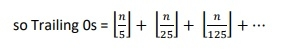# C/C++ Program to Count trailing zeroes in factorial of a number?

CC++Server Side ProgrammingProgramming

Here we will see how to calculate the number of trailing 0s for the result of factorial of any number. So if the n = 5, then 5! = 120. There is only one trailing 0. For 20!, it will be 4 zeros as 20! = 2432902008176640000.

The easiest approach is just calculating the factorial and count the 0s. But this approach fails for large value of n. So we will follow another approach. The trailing zeros will be there, if the prime factors are 2 and 5. If we count the 2s and 5s we can get the result. For that we will follow this rule.

Trailing 0s = Count of 5s in prime factors of factorial(n)## Algorithm

#### countTrailingZeros(n)

begin
count := 0
for i := 5, (n/i) >= 1, increase i := i * 5, do
count := count + (n / i)
done
return count;
end

## Example

#include <iostream>
#include <cmath>
#define MAX 20
using namespace std;
int countTrailingZeros(int n) {
int count = 0;
for (int i = 5; n / i >= 1; i *= 5)
count += n / i;
return count;
}
main() {
int n = 20;
cout << "Number of trailing zeros: " << countTrailingZeros(n);
}

## Output

Number of trailing zeros: 4